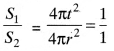# MP Board Class 9th Maths Solutions Chapter 13 Surface Areas and Volumes Ex 13.4

## MP Board Class 9th Maths Solutions Chapter 13 Surface Areas and Volumes Ex 13.4

Assume π = $$\frac{22}{7}$$, unless stated otherwise.

Question 1.
Find the surface area of a sphere of radius:

(i) 10.5 cm
(ii) 5.6 cm
(iii) 14 cm

Solution:
(i) Here r = 10.5 cm
∴ Surface area of the sphere = 4πr2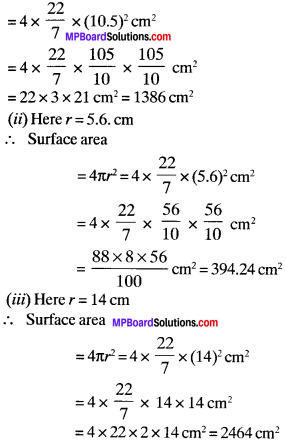Question 2.
Find the surface area of a sphere of diameter:

(i) 14 cm
(ii) 21 cm
(Hi) 3.5 m

Solution:
(i) Here, Diameter = 14 cm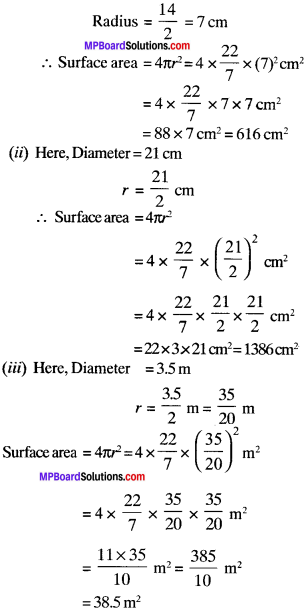Question 3.
Find the total surface area of a hemisphere of radius 10 cm. (Use π = 3.14)
Solution:
Here, radius (r) = 10 cm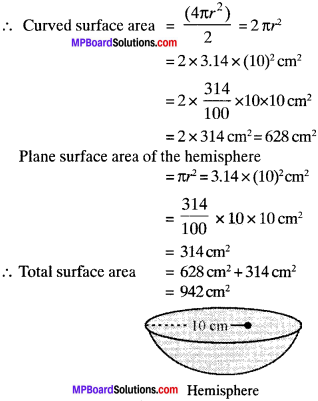Question 4.
The radius of a spherical balloon increases from 7 cm to 14 cm as air is being pumped into it. Find the ratio of surface areas of the balloon in the two cases.
Solution:
Initial radius of the balloon, r1 = 7 cm
Final radius of the balloon, r2 = 14 cm
Initial surface area of the balloon = S1
Final surfacg area of the balloon = S2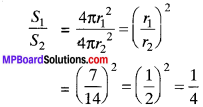S1 : S2 = 1 : 4

Question 5.
A hemispherical bowl made of brass has inner diameter 10.5 cm. Find the cost of tin-plating it on the inside at the rate of ₹ 16 per 100 cm2.
Solution:
d = 10.5 cm
r =5.25 cm
Rate of tinplating = $$\frac{16}{100}$$ = ₹ 0.16 per cm2
Inner curved surface area of bow l = 2 πr2
ICSA = 2 x $$\frac{22}{7}$$ x 5.25 x 5.25 = 173.25 cm2
Cost of tin-plating = Rate x ICSA
= 0.16 x 173.25 = ₹ 27.72Question 6.
Find the radius of a sphere whose surface area is 154 cm2.
Solution:
Surface area of sphere = 4 πr2
154 = 4 x $$\frac{22}{7}$$ x r2
$$\frac{154×7}{4×22}$$ = r2
⇒ r2 = 3.5 cm.

Question 7.
The diameter of the moon is approximately one-fourth of the diameter of the earth – Find the ratio of their surface areas.
Solution:
d1 = $$\frac{1}{4}$$d2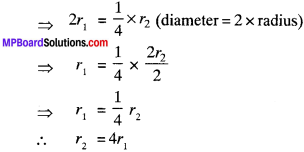Let .S1, and .S2, be the surface area of moon and earth respectively.
S1 = 4 πr12
S2 = 4 πr22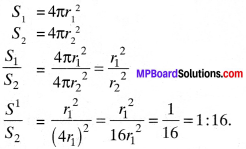Question 8.
A hemispherical bowl is made of steel, 0.25 cm thick. The inner radius of the bowl is 5 cm. Find the outer curved surface area of the bowl.
Solution:
r1 = 5 cm
t (thickness) = 0.25 cm
r2 = r1 + t
= 5 + 0.25 = 5.25 cm
OCSA of bowl = 2x $$\frac{22}{7}$$ x 5.25 x 5.25
= $$\frac{44}{7}$$ x 5.25 x 5.25 = 173.25 cm2Question 9.
A right circular cylinder just encloses a sphere of radius r (see Fig.). Find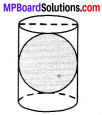1. surface area of the sphere.
2. curved surface area of the cylinder.
3. ratio of the areas obtained in (i) and (ii).

Solution:
As the sphere just encloses a right circular cylinder, the height of the cylinder is equal to the diameter of the sphere.
∴ Height of cylinder h = 2r
where r is the radius of sphere

1. Surface area of sphere S1 = 4πr2

2. CSA of a cylinder,= 2πrh
= 2πr x 2r = 4πr2

3.